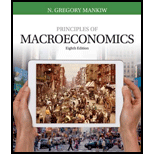# Let’s consider the effects of inflation in an economy composed of only two people: Bob, a bean farmer, and Rita, a rice farmer. Bob and Rita both always consume equal amounts of rice and beans. In 2016, the price of beans was $1 and the price of rice was$3. a. Suppose that in 2017 the price of beans was $2 and the price of rice was$6. What was inflation? Was Bob better off, worse off, or unaffected by the changes in prices? What about Rita? b. Now suppose that in 2017 the price of beans was $2 and the price of rice was$4. What was inflation? Was Bob better off, worse off, or unaffected by the changes in prices? What about Rita? c. Finally, suppose that in 2017 the price of beans was $2 and the price of rice was$1.50. What was inflation? Was Bob better off, worse off, or unaffected by the changes in prices? What about Rita? d. What matters more to Bob and Rita—the overall inflation rate or the relative price of rice and beans?### Principles of Macroeconomics (Mind...

8th Edition
N. Gregory Mankiw
Publisher: Cengage Learning
ISBN: 9781305971509### Principles of Macroeconomics (Mind...

8th Edition
N. Gregory Mankiw
Publisher: Cengage Learning
ISBN: 9781305971509

#### Solutions

Chapter
Section
Chapter 17, Problem 5PA
Textbook Problem
55 views

## Let’s consider the effects of inflation in an economy composed of only two people: Bob, a bean farmer, and Rita, a rice farmer. Bob and Rita both always consume equal amounts of rice and beans. In 2016, the price of beans was $1 and the price of rice was$3.a. Suppose that in 2017 the price of beans was $2 and the price of rice was$6. What was inflation? Was Bob better off, worse off, or unaffected by the changes in prices? What about Rita?b. Now suppose that in 2017 the price of beans was $2 and the price of rice was$4. What was inflation? Was Bob better off, worse off, or unaffected by the changes in prices? What about Rita?c. Finally, suppose that in 2017 the price of beans was $2 and the price of rice was$1.50. What was inflation? Was Bob better off, worse off, or unaffected by the changes in prices? What about Rita?d. What matters more to Bob and Rita—the overall inflation rate or the relative price of rice and beans?

Expert Solution

Subpart (a):

To determine
Relative price and inflation.

### Explanation of Solution

When the price of beans in 2017 is $2 and rice is$6, the inflation rate in year 2017 is calculated as follows:

Inflation rate=Price of of one unit of goods2017Price of of one unit of goods2016Price of of one unit of goods2016×100=(2+6</

Expert Solution

Subpart (b):

To determine
Relative price and inflation.

Expert Solution

Subpart (c):

To determine
Relative price and inflation.

Expert Solution

Subpart (d):

To determine
Relative price and inflation.

### Want to see the full answer?

Check out a sample textbook solution.See solution

### Want to see this answer and more?

Bartleby provides explanations to thousands of textbook problems written by our experts, many with advanced degrees!

See solution